# Texas Go Math Grade 5 Lesson 1.5 Answer Key Round Decimals

Refer to our Texas Go Math Grade 5 Answer Key Pdf to score good marks in the exams. Test yourself by practicing the problems from Texas Go Math Grade 5 Lesson 1.5 Answer Key Round Decimals.

## Texas Go Math Grade 5 Lesson 1.5 Answer Key Round Decimals

Unlock the Problem

The Gold Frog of South America is one of the smallest frogs in the world. It is 0.386 of an inch long. What is this length rounded to the nearest hundredth of an inch?

• Underline the length of the Gold Frog.
• Is the frog’s length about the same as the length or the width of a large paper clip?

One Way
Use a place-value chart.

• Write the number in a place-value chart and circle the digit in the place value to which you want to round.
• In the place-value chart, underline the digit to the right of the place to which you are rounding.
• If the digit to the right is less than 5, the digit in the place value to which you are rounding stays the same.
• If the digit to the right is 5 or greater, the digit in the rounding place increases by 1.
• Drop the digits after the place to which you are rounding.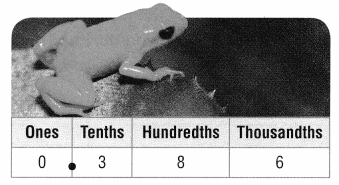Think: Does the digit in the rounding place stay the same or increase by 1?

So, to the nearest hundredth of an inch, a Gold Frog is about 0.39 of an inch long.

Another Way

Use place value.
The Little Grass Frog is the smallest frog in North America. It is 0.437 of an inch long

A. What is the length of the frog to the nearest hundredth of an inch?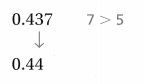So, to the nearest hundredth of an inch,
The frog is about 0.44 of an inch long.

B. What is the length of the frog to the nearest tenth of an inch?So, to the nearest tenth of an inch,
The frog is about 0.4 of an inch long.

Share and Show

Write the place value of the underlined digit. Round each number to the place of the underlined digit.

Question 1.
0.673
The given decimal number is: 0.673
Now,
The representation of the given number in the place value chart is:So,
From the given place value chart,
The place value of 7 is: Hundredths
So,
The rounded number that is to the place of the underlined digit is: 0.67
Hence, from the above,
We can conclude that
The place value of 7 is: Hundredths
The rounded number that is to the place of the underlined digit is: 0.67

Question 2.
4.282
The given decimal number is: 4.282
Now,
The representation of the given number in the place value chart is:So,
From the given place value chart,
The place value of 2 is: Tenths
So,
The rounded number that is to the place of the underlined digit is: 4.2
Hence, from the above,
We can conclude that
The place value of 2 is: Tenths
The rounded number that is to the place of the underlined digit is: 4.2

Question 3.
12.917
The given decimal number is: 12.917
Now,
The representation of the given number in the place value chart is: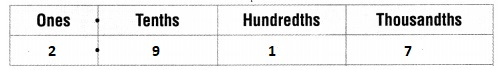So,
From the given place value chart,
The place value of 2 is: Ones
So,
The rounded number that is to the place of the underlined digit is: 13
Hence, from the above,
We can conclude that
The place value of 2 is: Ones
The rounded number that is to the place of the underlined digit is: 13

Name the place value to which each number was rounded.

Question 4.
0.982 to 0.98
The given decimal number is: 0.982
Now,
The representation of the given number in the place value chart is: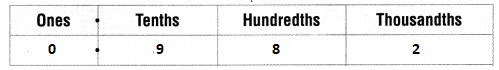Now,
When we observe that above place value chart,
We can observe that
The thousandths place is less than 5
Hence, from the above,
We can conclude that
The place value that the given number was rounded is: Thousandths

Question 5.
3.695 to 4
The given decimal number is: 3.695
Now,
The representation of the given number in the place value chart is:Now,
When we observe that above place value chart,
We can observe that
The tenths place is greater than 5
Hence, from the above,
We can conclude that
The place value that the given number was rounded is: Tenths

Question 6.
7.486 to 7.5
The given decimal number is: 7.486
Now,
The representation of the given number in the place value chart is:Now,
When we observe that above place value chart,
We can observe that
The hundredths place is greater than 5
Hence, from the above,
We can conclude that
The place value that the given number was rounded is: Hundredths

Problem Solving

Write the place value of the underlined digit. Round each number to the place of the underlined digit.

Question 7.
0.592
The given decimal number is: 0.592
Now,
The representation of the given number in the place value chart is: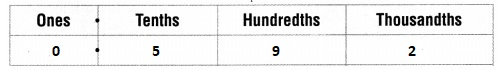Now,
From the above place value chart,
The place value of 5 is: 5 tenths
So,
The rounded number that is to the place of the underlined digit for the given number is: 0.6
Hence, from the above,
We can conclude that
The place value of 5 is: 5 tenths
The rounded number that is to the place of the underlined digit for the given number is: 0.6

Question 8.
6.518
The given decimal number is: 6.518
Now,
The representation of the given number in the place value chart is: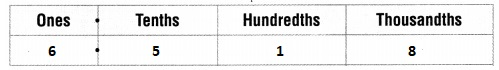Now,
From the above place value chart,
The place value of 6 is: 6 ones
So,
The rounded number that is to the place of the underlined digit for the given number is: 7
Hence, from the above,
We can conclude that
The place value of 6 is: 6 ones
The rounded number that is to the place of the underlined digit for the given number is: 7

Question 9.
0.809
The given decimal number is: 0.809
Now,
The representation of the given number in the place value chart is: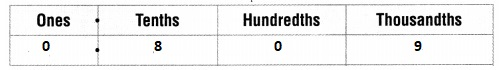Now,
From the above place value chart,
The place value of 0 is: 0 hundredths
So,
The rounded number that is to the place of the underlined digit for the given number is: 0.8
Hence, from the above,
We can conclude that
The place value of 0 is: 0 hundredths
The rounded number that is to the place of the underlined digit for the given number is: 0.8

Round 16.748 to the place named.

Question 10.
tenths __________
The given number is: 16.748
Now,
The representation of the given number in the place value chart is: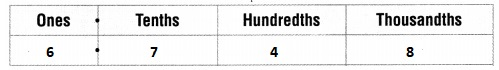Now,
From the given place value chart,
We can observe that
The tenths place value is greater than 5
Hence, from the above,
We can conclude that
The rounded number that is to the nearest tenth for the given number is: 16.8

Question 11.
hundredths __________
The given number is: 16.748
Now,
The representation of the given number in the place value chart is:Now,
From the given place value chart,
We can observe that
The hundredths place value is less than 5
Hence, from the above,
We can conclude that
The rounded number that is to the nearest hundredth for the given number is: 16.74

Question 12.
ones _____________
The given number is: 16.748
Now,
The representation of the given number in the place value chart is:Now,
From the given place value chart,
We can observe that
The ones place value is greater than 5
Hence, from the above,
We can conclude that
The rounded number that is to the nearest one for the given number is: 20

Question 13.
Explain why any number less than 12.5 and greater than or equal to 11.5 would round to 12 when rounded to the nearest whole number.
Let the number be x
Now,
We know that,
When the last digit of a number is greater than 5, then it will be rounded to the next whole number
When the last digit of a number is less than 5, then it will be rounded to the nearest previous whole number
So,
If x = 11.6, then
x = 12 when x > 11.5 and x < 12.5
Hence, from the above,
We can conclude that
When the last digit of a number is greater than 5, then it will be rounded to the next whole number
When the last digit of a number is less than 5, then it will be rounded to the nearest previous whole number

Question 14.
Write Math Explain what happens when you round 4.999 to the nearest tenth.
The given decimal number is: 4.999
Now,
The representation of 4.999 in the place value chart is:Now,
When we observe the tenth’s place value in the above lace value chart
We can observe that
The number is greater than 5
Now,
We know that,
When the last digit of a number is greater than 5, then it will be rounded to the next whole number
When the last digit of a number is less than 5, then it will be rounded to the nearest previous whole number
Hence, from the above,
We can conclude that
The rounded number for 4.999 when we rounded off to the nearest tenth to the whole number is: 5

Problem Solving

Use the table for 15-18.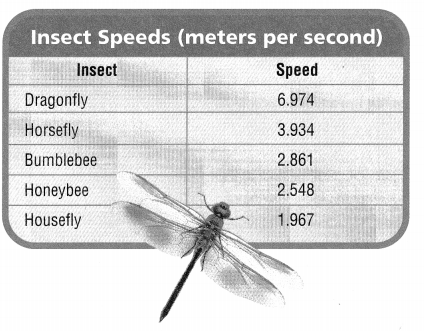Question 15.
The speeds of two insects, when rounded to the nearest whole number, are the same. Which two insects are they?
The given table is:Now,
From the given table,
We can say that
The rounded speeds nearest to the whole number of the different insects are:
Dragonfly      –         7 m/s
Horsefly         –         4 m/s
Bumblebee    –         3 m/s
Honeybee      –         3 m/s
Housefly        –          2 m/s
Hence, from the above,
We can conclude that
The  names of the insects that have the speeds of the two insects when rounded to the nearest to the whole number that are the same are: Bumblebee and Honeybee

Question 16.
What is the speed of the housefly rounded to the nearest hundredth?
The given table is:Now,
From the given table,
We can observe that
The speed of Housefly is: 1.967 m/s
Now,
The representation of the speed of housefly in the place value chart is: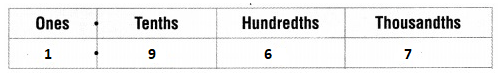Now,
From the above place value chart,
We can say that
The speed of the housefly when rounded to the nearest hundredth is: 1.97 m/s
Hence, from the above,
We can conclude that
The speed of the housefly when rounded to the nearest hundredth is: 1.97 m/s

Question 17.
The speed of an insect is about 3.9 meters per second. Which insect could it be?
It is given that
The speed of an insect is about 3.9 meters per second
Now,
The given table is:Now,
From the given table,
We can observe that
The speed of the horsefly is: 3.934 m/s
Now,
When we rounded off the speed of horsefly to the nearest hundredth,
The speed of horsefly will be: 3.9 m/s
Hence, from the above,
We can conclude that
The insect that has the speed of 3.9 m/s is: Horsefly

Question 18.
H.O.T. What’s the Error? Mark said that the speed of a dragonfly rounded to the nearest tenth was 6.9 meters per second. Is he correct? If not, what is his error?
It is given that
Mark said that the speed of a dragonfly rounded to the nearest tenth was 6.9 meters per second.
Now,
The given table is:Now,
From the given table,
We can observe that
The speed of dragonfly is: 6.974 m/s
Now,
When we round off the speed of dragonfly to the nearest tenth,
The speed of dragonfly will be: 6.9 m/s
Hence, from the above,
We can conclude that
Mark is correct

Question 19.
H.O.T. Multi-Step A rounded number for the speed of an insect is 5.67 meters per second. What are the fastest and slowest speeds to the thousandths that could round to 5.67? Explain.
It is given that
A rounded number for the speed of an insect is 5.67 meters per second.
Now,
When we round off the given speed of insect to the nearest whole number,
The speed of insect will become: 6 m/s
When we round off the given speed of insect to the hundredth,
The speed of insect will become: 5.7 m/s
Hence, from the above,
We can conclude that
The fastest speed is: 6 m/s
The slowest speed is: 5.7 m/s

Question 20.
Chef Round uses 1.257 kilograms of meat for his famous meatballs. There are four packages at the market. Which amount is closest to 1.257 kilograms?
A. 1.26 kg
B. 1.2 kg
C. 1.3 kg
D. 1.35 kg
It is given that
Chef Round uses 1.257 kilograms of meat for his famous meatballs. There are four packages at the market.
Now,
The given number is: 1.257
When we round off the given number to the nearest thousandth,
The number will be: 1.26
Hence, from the above,
We can conclude that
The amount that is closest to 1.257 kilograms is: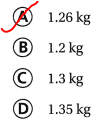Question 21.
Representations The table shows the neck lengths for four giraffes in the Long-Necked Friends Sanctuary. Which two giraffes have necks that are the same length when rounded to the nearest tenth?A. Spot and Stretch
B. Mittens and Spot
C. Mittens and Stretch
D. Spot and Zippy
It is given that
The table shows the neck lengths for four giraffes in the Long-Necked Friends Sanctuary.
Now,
The given table is:Now,
From the given table,
We can say that
The length of the necks when rounded off to the nearest tenth is:
Mittens            –               5.6 feet
Spot                 –               5.7 feet
Stretch             –               5.4 feet
Zippy               –               5.7 feet
So,
The two giraffes that have the same neck length are: Spot and Zippy
Hence, from the above,
We can conclude that
The two giraffes that have the same neck lengths when rounded to the nearest tenth are: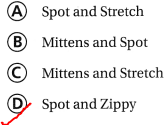Question 22.
Multi-Step Janis sells friendship bracelets at a fair. She has three bracelets that are 16.53 cm, 16.755 cm, and 16.55 cm long. She wants to label the lengths to the nearest tenth of a cm. In order, how should she label the bracelets?
A. 16.5 cm, 16.76 cm, 16.6 cm
B. 16.6 cm, 16.7 cm, 16.5 cm
C. 16.5 cm, 16.8 cm, 16.6 cm
D. 16.3 cm, 16.5 cm, 16.5 cm
It is given that
Janis sells friendship bracelets at a fair. She has three bracelets that are 16.53 cm, 16.755 cm, and 16.55 cm long
Now,
The given numbers are: 16.53, 16.755, and 16.55
So,
The given numbers when rounded to the nearest tenth will become: 16.5, 16.76, and 16.6
So,
The order of the numbers from the greatest to the least are: 16.76, 16.6, and 16.5
The order of the numbers from the least to the greatest are: 16.5, 16.6, and 16.76
Hence, from the above,
We can conclude that
The order that Janis should label the bracelets are:Texas Test Prep

Question 23.
To which place value is the number rounded? 6.706 to 6.71
A. ones
B. tenths
C. hundredths
D. thousandths
The given decimal number is: 6.706
Now,
The representation of 6.706 in the place value chart is:Now,
From the above place value chart,
We can say that
The given rounded can be rounded to thousandths place to become 6.71
Hence, from the above,
We can conclude that
The place value that the given number is rounded will be: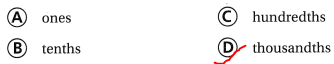### Texas Go Math Grade 5 Lesson 1.5 Homework and Practice Answer Key

Write the place value of the underlined digit. Round each number to the place of the underlined digit.

Question 1.
0.782
The given decimal number is: 0.782
Now,
The representation of the given number in the place value chart is: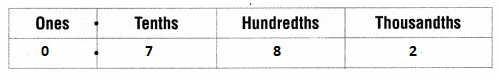Now,
From the above place value chart,
The place value of 7 is: 7 tenths
So,
The rounded number that is to the place of the underlined digit for the given number is: 0.8
Hence, from the above,
We can conclude that
The place value of 7 is: 7 tenths
The rounded number that is to the place of the underlined digit for the given number is: 0.8

Question 2.
4.071
The given decimal number is: 4.071
Now,
The representation of the given number in the place value chart is:Now,
From the above place value chart,
The place value of 7 is: 7 hundredths
So,
The rounded number that is to the place of the underlined digit for the given number is: 4.07
Hence, from the above,
We can conclude that
The place value of 7 is: 7 hundredths
The rounded number that is to the place of the underlined digit for the given number is: 4.07

Question 3.
21.939
The given decimal number is: 21.939
Now,
The representation of the given number in the place value chart is:Now,
From the above place value chart,
The place value of 1 is: 1 ones
So,
The rounded number that is to the place of the underlined digit for the given number is: 22
Hence, from the above,
We can conclude that
The place value of 1 is: 1 ones
The rounded number that is to the place of the underlined digit for the given number is: 22

Question 4.
8.155
The given decimal number is: 8.155
Now,
The representation of the given number in the place value chart is: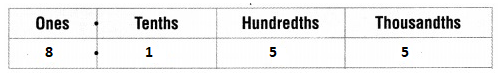Now,
From the above place value chart,
The place value of 1 is: 1 tenth
So,
The rounded number that is to the place of the underlined digit for the given number is: 8.16
Hence, from the above,
We can conclude that
The place value of 1 is: 1 tenth
The rounded number that is to the place of the underlined digit for the given number is: 8.16

Question 5.
34.09
The given decimal number is: 34.09
Now,
The representation of the given number in the place value chart is:Now,
From the above place value chart,
The place value of 3 is: 3 tens
So,
The rounded number that is to the place of the underlined digit for the given number is: 34
Hence, from the above,
We can conclude that
The place value of 3 is: 3 tens
The rounded number that is to the place of the underlined digit for the given number is: 34

Question 6.
4.276
The given decimal number is: 4.276
Now,
The representation of the given number in the place value chart is:Now,
From the above place value chart,
The place value of 7 is: 7 hundredths
So,
The rounded number that is to the place of the underlined digit for the given number is: 4.28
Hence, from the above,
We can conclude that
The place value of 7 is: 7 hundredths
The rounded number that is to the place of the underlined digit for the given number is: 4.28

Round 8.293 to the place named.

Question 7.
hundredths ___________
The given number is: 8.293
Now,
The representation of 8.293 in the place value chart is:Now,
From the above place value chart,
We can say that
The rounded value of 8.293 nearest to the place value of hundredths is: 8.29
Hence, from the above,
We can conclude that
The rounded value of 8.293 nearest to the place value of hundredths is: 8.29

Question 8.
tenths ______________
The given number is: 8.293
Now,
The representation of 8.293 in the place value chart is:Now,
From the above place value chart,
We can say that
The rounded value of 8.293 nearest to the place value of tenths is: 8.3
Hence, from the above,
We can conclude that
The rounded value of 8.293 nearest to the place value of tenths is: 8.3

Question 9.
ones ____________
The given number is: 8.293
Now,
The representation of 8.293 in the place value chart is:Now,
From the above place value chart,
We can say that
The rounded value of 8.293 nearest to the place value of ones is: 8
Hence, from the above,
We can conclude that
The rounded value of 8.293 nearest to the place value of ones is: 8

Round 12.462 to the place named.

Question 10.
tenths __________
The given number is: 12.462
Now,
The representation of 8.293 in the place value chart is:Now,
From the above place value chart,
We can say that
The rounded value of 12.462 nearest to the place value of tenths is: 12.5
Hence, from the above,
We can conclude that
The rounded value of 12.462 nearest to the place value of tenths is: 12.5

Question 11.
hundredths ___________
The given number is: 12.462
Now,
The representation of 8.293 in the place value chart is:Now,
From the above place value chart,
We can say that
The rounded value of 12.462 nearest to the place value of hundredths is: 12.46
Hence, from the above,
We can conclude that
The rounded value of 12.462 nearest to the place value of hundredths is: 12.46

Question 12.
ones _____________
The given number is: 12.462
Now,
The representation of 8.293 in the place value chart is:Now,
From the above place value chart,
We can say that
The rounded value of 12.462 nearest to the place value of ones is: 12
Hence, from the above,
We can conclude that
The rounded value of 12.462 nearest to the place value of ones is: 12

Problem Solving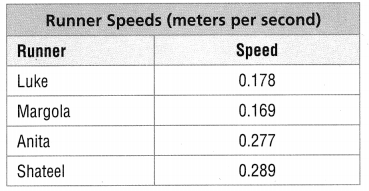Question 13.
What is Anita’s speed rounded to the nearest hundredth?
The given table is:Now,
From the given table,
We can observe that
Anita’s speed is: 0.277 m/s
Now,
The value of Anita’s speed when rounded to the nearest hundredth will become: 0.28 m/s
Hence, from the above,
We can conclude that
Anita’s speed that is rounded to the nearest hundredth is: 0.28 m/s

Question 14.
The speeds of two runners when rounded to the nearest tenth are the same. Which two runners are they?
The given table is:Now,
From the given table,
We can observe that
The values of runners speeds when rounded to the nearest tenth will be:
Luke             –            0.2 m/s
Margola       –            0.2 m/s
Anita            –            0.28 m/s
Shateel         –            0.30 m/s
So,
From the above,
We can observe that
The speed of the two runners that have the same speed when rounded to the nearest tenth are: Luke and Margola
Hence, from the above,
We can conclude that
The speed of the two runners that have the same speed when rounded to the nearest tenth are: Luke and Margola

Lesson Check

Question 15.
To which place value is the number rounded? 8.293 to 8.29
A. ones
B. tenths
C. hundredths
D. thousandths
The given decimal number is: 8.293
Now,
The representation of 8.293 in the place value chart is:So,
From the above place value chart
We can observe that
The number is rounded at the thousandths place
Hence, from the above,
We can conclude that
The place value that the given number is rounded is: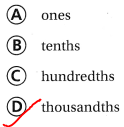Question 16.
To which place value is the number rounded? 0.799 to 0.8
A. ones
B. tenths
C. hundredths
D. thousandths
The given decimal number is: 0.799
Now,
The representation of 0.799 in the place value chart is:So,
From the above place value chart
We can observe that
The number is rounded at the hundredths place
Hence, from the above,
We can conclude that
The place value that the given number is rounded is:Question 17.
Which number is 5.389 rounded to the nearest hundredth?
A. 5.4
B. 5.38
C. 5.39
D. 5.399
The given decimal number is: 5.389
Now,
The representation of 5.389 in the place value chart is: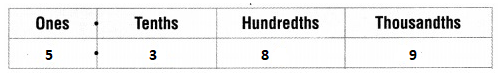Now,
From the above place value chart,
We can say that
The value of 5.389 when rounded to the nearest hundredth is: 5.39
Hence, from the above,
We can conclude that
The number that is to the nearest hundredth for 5.389 is: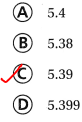Question 18.
Which number is 7.323 rounded to the nearest tenth?
A. 7.3
B. 7.4
C. 7.32
D. 7.33
The given decimal number is: 7.323
Now,
The representation of 7.323 in the place value chart is: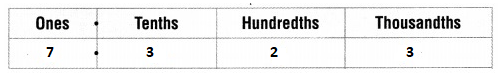Now,
From the above place value chart,
We can say that
The value of 7.323 when rounded to the nearest tenth is: 7.3
Hence, from the above,
We can conclude that
The number that is to the nearest tenth for 7.323 is: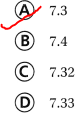Question 19.
A baker uses 2.327 kilograms of blueberries for muffins. There are four packages at the fruit market. Which amount is closest to 2.327 kilograms?
A. 2.3 kg
B. 2.42 kg
C. 2.33 kg
D. 2.4 kg
It is given that
A baker uses 2.327 kilograms of blueberries for muffins. There are four packages at the fruit market
Now,
The representation of 2.327 in the place value chart is: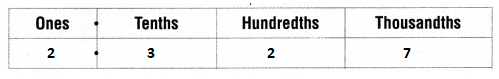Now,
From the above place value chart,
We can say that
The number that is nearest to 2.327 is: 2.33
Hence, from the above,
We can conclude that
The amount that is closest to 2.327 kilograms is: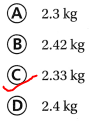Question 20.
Multi-Step The chart shows the length of each student’s pencil. Which person has a pencil length that can be rounded up to the nearest tenth of an inch?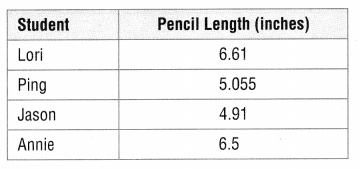A. Lori
B. Ping
C. Jason
D. Annie
It is given that
The chart shows the length of each student’s pencil.
Now,
The given table is:Now,
From the given table,
We can observe that
The values of  pencil lengths of Lori, Ping, and Jason are represented in hundredths whereas the value of pencil length for Annie is represented in tenths only
Hence, from the above,
We can conclude that
The person that has a pencil length that can be rounded up to the nearest tenth of an inch is: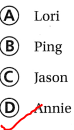Scroll to Top